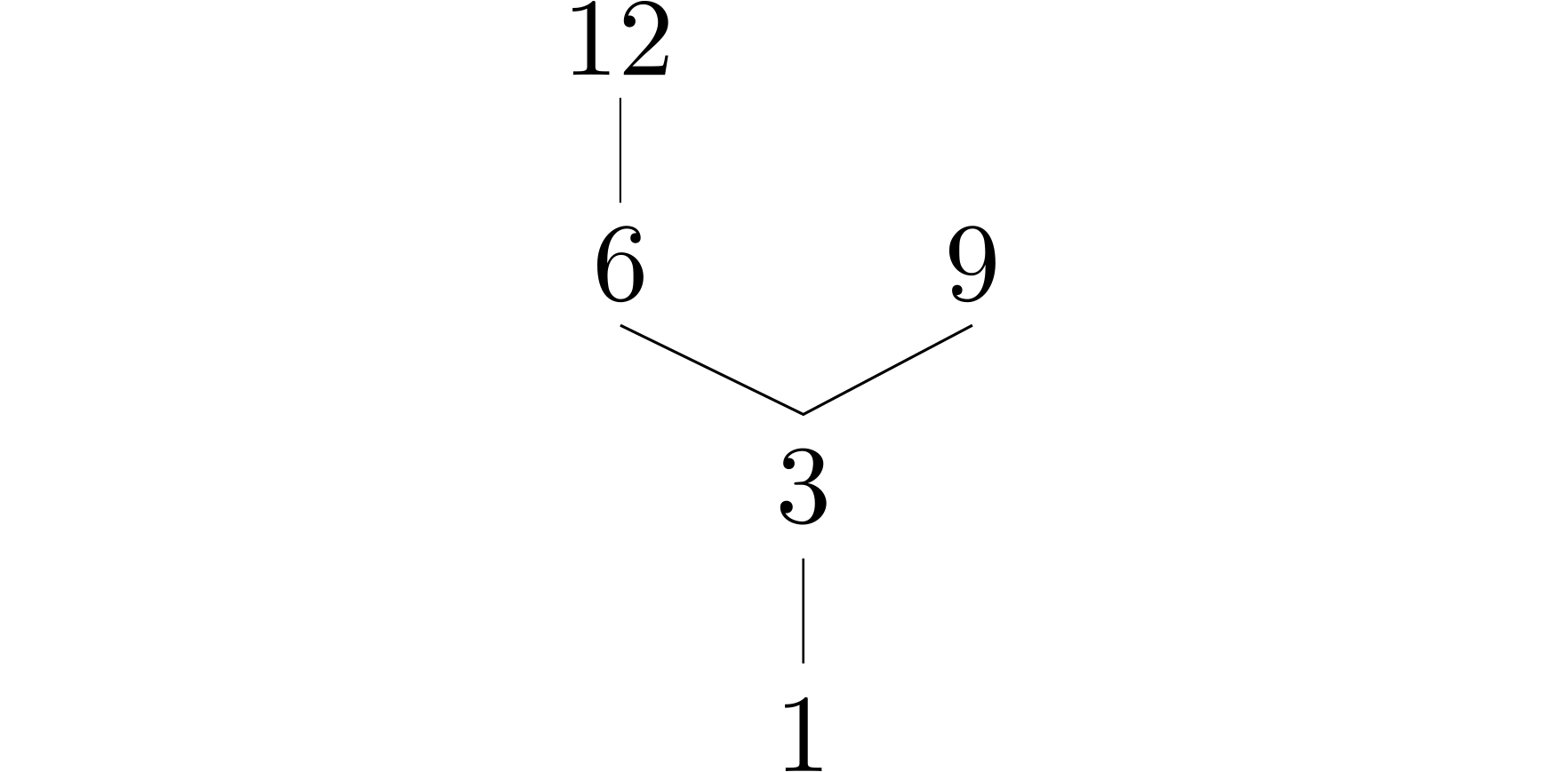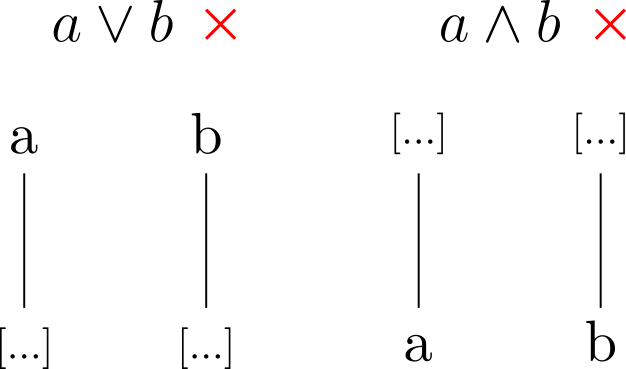Continuing with Discrete Mathematics (because I am too lazy to create nice graphics for Linear Algebra right now), we shall examine the following rather simple problem:

Prove or disprove: $(\lbrace 1,3,6,9,12\rbrace, \mid)$ is a lattice. (l06, 4c)

Let’s quickly recall what it means for a poset to be a lattice: A poset in which every pair of elements has a meet (= greatest lower bound) and a join (= least upper bound) is called a lattice.A visualization of the poset as a Hasse diagram would look like the image above. Now, as an example, let us try and pick any pair of elements, say $3$ and $6$. Then, the upper bounds of this pair are all elements $x$ such that $x \ge 3$ and $x \ge 6$. As one can easily find out by looking at the image, the upper bounds of $3$ and $6$ in this poset are $6$ and $12$. Thus, the lowest upper bound of $3$ and $6$, so the join, is $6$. As you may recall, an alternative notation for the join is $3 \lor 6 = 6$. The same applies dually for greatest lower bounds.

Now, the question remains if every pair of elements has such a join and a meet. In this example, we can clearly see that both $\lbrace 6, 9 \rbrace$ and $\lbrace 9, 12$ have no common upper bound. Therefore, we can already conclude that this poset is not a lattice. More generally, if a finite poset does not have a least and a greatest element, it cannot be a lattice as visible in this figure:The opposite implication is (sadly, I guess) not true though: Just because a poset has a least and a greatest element does not mean that is must be a lattice. Can you find a counterexample for this case?

Therefore, we must manually check all pairs to be sure that we indeed have a lattice. Since the cardinality of the poset in these exercises is usually quite small, this doesn’t take long, especially if you quickly sketch the Hasse diagram.

Practice: Is $(\lbrace 1, 2, 3, 4, 6, 12 \rbrace ; \mid)$ a lattice?

Tomorrow, I hope to finally publish a Linear Algebra post, with some nice graphics regarding basis transforms, dictum meum pactum.